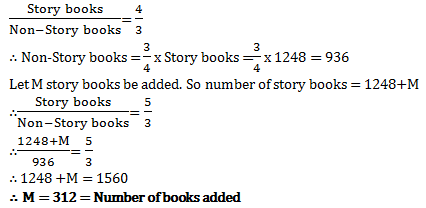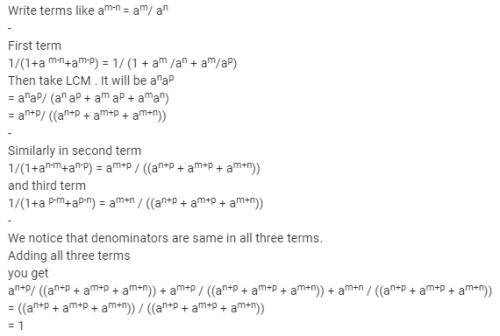# Test: Ratio And Proportion, Indices, Logarithms - 4

## 40 Questions MCQ Test Quantitative Aptitude for CA CPT | Test: Ratio And Proportion, Indices, Logarithms - 4

Description
Attempt Test: Ratio And Proportion, Indices, Logarithms - 4 | 40 questions in 40 minutes | Mock test for CA Foundation preparation | Free important questions MCQ to study Quantitative Aptitude for CA CPT for CA Foundation Exam | Download free PDF with solutions
QUESTION: 1

Solution:
QUESTION: 2

Solution:
QUESTION: 3

### The number which has the same ratio to 26 that 6 has to 13 is

Solution:
QUESTION: 4

If (5x-3y)/(5y-3x) =3/4 , the value of x:y is

Solution:
QUESTION: 5

The mean proportional between 12x2 and 27y2 is

Solution:
QUESTION: 6

If x:y=z:w=2.5/1.5, the value of (x+z)/(y+w) is 0

Solution:
QUESTION: 7

The mean proportional between 25,81 is

Solution:
QUESTION: 8

The fourth proportional to 4,6,8 is

Solution:
QUESTION: 9

If A:B=3:2 and B:C=3:5, then A:B:C is

Solution:
QUESTION: 10

If a/3=b/4=c/7, then a+b+c/c is

Solution:

a/ 3 = b/ 4 = c/7

a/c = 3/ 7 ; b/ c = 4/7

(a+b+c) / c ⇒ a/ c+ b/ c + 1

⇒ 3/7 + 4/7+ 1

⇒ 1 + 1

⇒ 2

QUESTION: 11

Division of Rs. 750 into 3 parts in the ratio 4:5:6 is

Solution:
QUESTION: 12

If A = B/2 = C/5, then A:B:C is

Solution:
QUESTION: 13

The third proportional to 12,18 is

Solution:

Let the third proportion be x

12 : 18 = 18 : x

x = 18*(18/12)

x = 18*(3/2)

x = 54/2

x = 27

QUESTION: 14

In a library, the ratio of number of story books to that of non-story books was 4:3 and total number of story books was 1248. When some more story books were bought, the ratio became 5:3. Find the number of story books bought.

Solution:QUESTION: 15

If x/y=z/w, implies y/x=w/z, then the process is called

Solution:
QUESTION: 16

If u/v=w/p, then (u-v)/(u+v)=(w-p)/(w+p). The process is called

Solution:
QUESTION: 17

What is the fourth proportional to the numbers 2, 5, 8.​

Solution:
QUESTION: 18

If p/q=r/s=p-r/q-s, the process is called

Solution:
QUESTION: 19

The sum of the ages of 3 persons is 150 years. 10 years ago their ages were in the ratio 7:8:9. Their present ages are

Solution:
QUESTION: 20

Two numbers are in the ratio 3:4; if 6 be added to each terms of the ratio, then the new ratio will be 4:5, then the numbers are

Solution:
QUESTION: 21

If a/b=c/d, implies (a+b)/(a-b) = (c+d)/(c-d), the process is called

Solution:
QUESTION: 22

12, 16, *, 20 are in proportion. Then* is

Solution:
QUESTION: 23

The numbers 14, 16, 35, 42 are not in proportion. The fourth term for which they will be in proportion is

Solution:
QUESTION: 24

4x-1/4 is expressed as

Solution:
QUESTION: 25

Xa-b x Xb-c x Xc-a is equal to

Solution:
QUESTION: 26

The value of 2(256)-1/8 is

Solution:
QUESTION: 27

Which is True?

Solution:
QUESTION: 28

The value of 4/(32)1/5 is

Solution:
QUESTION: 29

If x1/p = y 1/q = z1/r and xyz =1 , then the value of p+q+r is

Solution:
QUESTION: 30

The value of (8/27)1/3 is

Solution:
QUESTION: 31

{(33)2 x (42)3 x (53)2}/{(32)3 x (43)2 x (52)3} is

Solution:
QUESTION: 32

2 ½ . 4 ¾ is equal to

Solution:

Explanation : 21/2 * 41/2

1 * 2

= 2 (positive integer)

QUESTION: 33

The simplified value of 16x-3y2 x 8-1x3y-2 is

Solution:
QUESTION: 34

The value of 2 x (32)1/5 is

Solution:
QUESTION: 35

The True option is

Solution:
QUESTION: 36

The value of ya-b x yb-cx yc-a x y-a-b is

Solution:
QUESTION: 37

The value of 81/3 is

Solution:
QUESTION: 38

On simplification, 1/(a+am-n + am-p) + 1/(1+an-m +an-p) + 1/(1+ap-m +ap-n) is equal to

Solution:QUESTION: 39

[{(2)1/2. (4)3/4. (8)5/6. (16)7/8. (32)9/10}4]3/25 is

Solution:
QUESTION: 40

If four numbers 1/2, 1/3, 1/5, 1/x are proportional then x is

Solution:Use Code STAYHOME200 and get INR 200 additional OFF Use Coupon Code